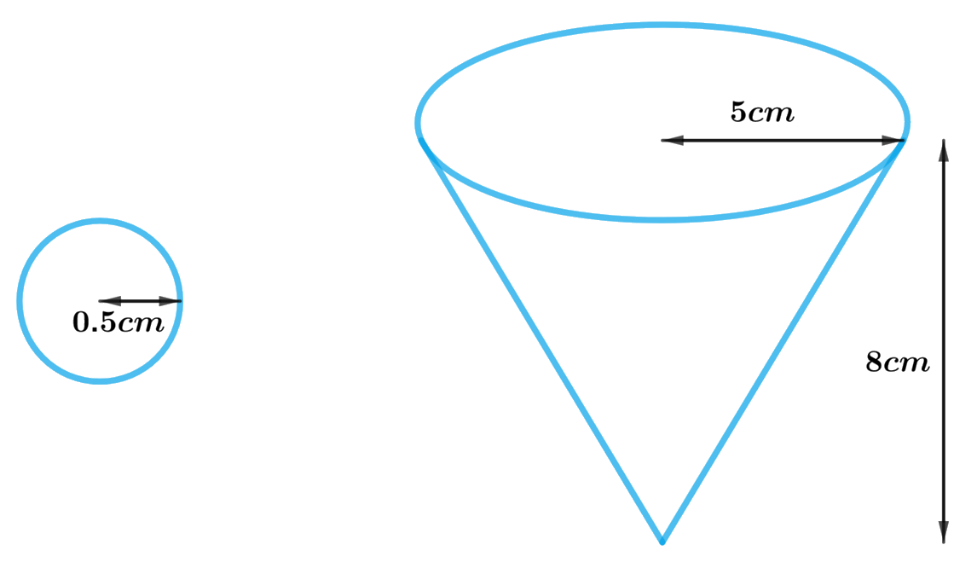# Ex.13.2 Q5 Surface Areas and Volumes Solution - NCERT Maths Class 10

Go back to  'Ex.13.2'

## Question

A vessel is in the form of an inverted cone. Its height is $$8\,\rm{cm}$$ and the radius of its top, which is open, is $$5\,\rm{cm}$$. It is filled with water up to the brim. When lead shots, each of which is a sphere of radius $$0.5\,\rm{cm}$$ are dropped into the vessel, one-fourth of the water flows out. Find the number of lead shots dropped in the vessel.

Video Solution
Surface Areas And Volumes
Ex 13.2 | Question 5

## Text Solution

What is known?

An open vessel is in the form of an inverted cone with height $$=8\,\rm{cm}$$ and radius of its top $$= 5\,\rm{cm}$$, which is filled with water up to the drum.

Spherical lead shots each of  radius $$=0.5\,\rm{cm},$$ are dropped into the vessel until then one-fourth of the water in the vessel flows out.

What is unknown?

Number of lead shots dropped into the vessel.

Reasoning:

Draw a figure of the vessel and lead shot to visualize itSince the water is filled up to the brim in the vessel

Volume of water in the vessel $$=$$ volume of the conical vessel

Also, on dropping a certain number of lead shots (sphere) into the vessel one-fourth of the water flows out.

Volume of all lead shots dropped in the vessel \begin{align} = \frac{1}{4} \times \end{align}Volume of water in the vessel

Hence,

Number of lead shots \begin{align} = \frac{1}{4} \times\end{align}volume of the water in the vessel$$\div$$ volume of each lead shot

We will find the volume of the water in the vessel and lead shot by using formulae;

Volume of the sphere\begin{align} = \frac{4}{3}\pi {r^3}\end{align}

where $$r$$ is the radius of the hemisphere

Volume of the cone\begin{align}= \frac{1}{3}\pi {R^2}h\end{align}

where $$R$$ and $$h$$ are the radius and height of the cone respectively

Steps:

Height of the conical vessel,$$h = 8 \rm cm$$

Radius of the conical vessel,$$R = 5 \rm cm$$

Radius of the spherical lead shot,$$r = 0.5 \rm cm$$

Number of lead shots\begin{align}= \frac{1}{4} \times\end{align} volume of the water in the vessel $$\div$$volume of each lead shot

\begin{align}&= \left( {\frac{1}{4} \times \frac{1}{3}\pi {R^2}h} \right) \div \left( {\frac{4}{3}\pi {r^3}} \right)\\&= \frac{{\pi {R^2}h}}{{12}} \times \frac{3}{{4\pi {r^3}}}\\&= \frac{{{R^2}h}}{{16{r^3}}}\\&= \frac{{5 \rm cm \times 5cm \times 8cm}}{{16 \times 0.5 \rm cm \times 0.5cm \times 0.5cm}}\\ &= 100\end{align}

Learn from the best math teachers and top your exams

• Live one on one classroom and doubt clearing
• Practice worksheets in and after class for conceptual clarity
• Personalized curriculum to keep up with school